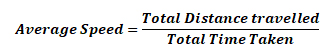Speed - Physics
22-07-2018    1652 times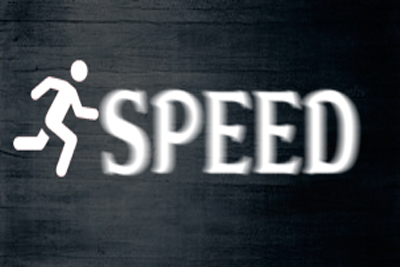### Speed

The distance travelled either a straight line or a circular line by a rigid body in a unit time is known as the speed of the rigid body.

Direction is not required for mention speed of a rigid body. It is a scalar quantity. A car is moving 20 kilometres / hour. Here 20 km / hour is the speed.

i.e. if a rigid body moves s distance in t second then the speed of the rigid body will be s/t.

### Units of the speed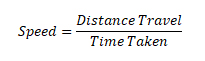MKS or IS method:metre / second (ms-1)

CGS method : centimetre / seconds (cm s-1)

FPS method : foot / seconds ( fs-1)

Kinds of speed

Speeds are divided into four types

1. Uniform speed: If the body is covering equal distance in equal interval of time in any direction, then that speed is known as uniform speed.

For Examples, if a car travels 60 km / HR. That is 1km / minute. Then we can say that the car has a uniform speed of 6okm / HR. Or 1 km / min.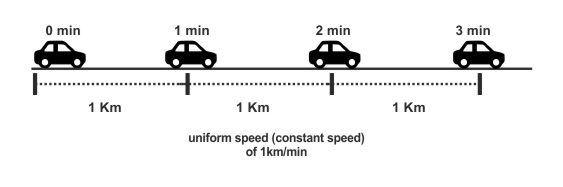1. Non-uniformed speed Or variable speed: If the body is covered unequal distance in equal interval of time in any direction, then that speed is known as non-uniform speed or variable speed.

For examples, if a car travels 30km in 30 minutes, 25 km in next 30 minutes, 20 km in next 30 minutes. Then we can say that the car has a non-uniform speed.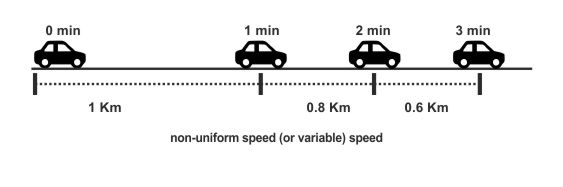1. Instantaneous Speed: If a rigid body changes its speed continuously with time, then the speed of the rigid body at any instant or any moment is known as instantaneous speed.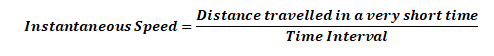1. Average Speed: When a body travel with a variable speed, then the average speed will be calculated by dividing the total distance cover by the body and the total time is taken.